TECH 4
 Racing fuel characteristics
For many racers, racing fuels are considered to be the ultimate high performance part. These fuels are
designed to help extract the last bit of torque and power from a racing engine. They can be blended for
applications ranging from a stock unmodified high performance engine to a full-blown "top fuel" race
engine. We will look at the different characteristics between some of these fuels.

The quality of racing fuel is much more consistent than standard pump gas, which racers refer to as
"
chemical soup".  In order to meet specifications, pump gas is blended in mass quantity and is made up of
several hundred hydrocarbon fractions. Although the power output is close to that of racing fuel, it's the
inconsistencies in power output that makes it difficult for engine tuners.  Since The Clean Air Act of 1990,
fuel chemists are now more than ever "tinkering" with their blends to meet EPA mandates for improved
combustion efficiency, better fuel economy and lower emissions.

It is important to first realize that all the horsepower an engine will ever produce is stored in the fuel. Since
this can be expressed in mathematical terms, engine power can be predicted.  The second law of
thermodynamics states that "heat cannot be completely converted into another form of energy" (e.g.
Mechanical work).

For most 4-stroke (otto-cycle) engines, there are thermal inefficiencies in the compression and expansion
processes.  Because of this, only a small percentage, typically less than 33%, of the resultant combustion
heat can be converted to mechanical force on the piston.

As racers, we are willing to burn just about anything through our cylinders to increase that percentage of
converted mechanical force.  Racing fuels can help extract the most mechanical force from an engine by
increasing its
combustion efficiency(CE) and delivering the most power to the piston.

When choosing a particular racing fuel for a specific engine, many factors need to be considered.
It is important to understand some of the basic properties of fuel that influence power and performance.

1.
Heat value (HV)- or the energy potential of the fuel can be expressed in British Thermal Units (BTUs)
per pound.
Octane (C8H18) has around 19,200 BTUs per pound while typical pump gas is around 18,400
(BTUs), with premium being slightly higher.  Higher (BTU) ratings will potentially yield more power at any
compression ratio or engine rpm.  As stated earlier, power potential can be predicted mathematically with
the basic equation 2,544.5 (BTUs) per hour =1 horsepower per hour. Therefore horsepower is directly
proportional to the amount of fuel that can be efficiently burned.

A comparison of heat values between three types of fuel looks like this.(sources may vary)

FIGURE 1   Specific Energy = Heat Value / AF Ratio.

In Figure 1: we first realize that (C8H18) has the highest (HV) at 19,200 (BTUs) per pound, but requires
15.1 pounds of air to consume that amount. This is determined by the
air:fuel (AF) ratio.

The (AF) ratio is considered to be
stoichiometric when a sufficient air mass is provided for complete
combustion (neither rich nor lean). The
oxygen (O2) mass is only 21% of the stoichiometric air mass, and
does not contribute to a (HV).  It only functions as an oxidizer that allows the fuel to complete the chemical
reactions of combustion while it releases its (HV).

The (HV) released during combustion is directly related to only the
hydrogen (H2) and carbon (C) bonds in
the fuel.  Once heat is added and the (H2) and (C) bonds reach ignition temperature, they are broken and
reformed with (O2) to form stronger bonds and complete the exothermic chemical reactions. They are the
complete formation of
water (H2O) and carbon dioxide (CO2), also known as "exhaust gases". The (H2O)
formation efficiency affects the heat range of the fuel.  If (H2O) forms as steam, it is considered to be the
lower (HV). If it forms as water vapor, additional heat can be released through evaporation/expansion and
is considered to be the higher (HV).

If we divide the (HV) of (C8H18) 19,200 by the (AF) ratio 15.1 we arrive at the
specific energy (SE) of the
fuel at 1,272 (BTUs). The (SE) is a more precise estimation of potential power. It determines the amount of
(BTUs) per pound of air.  The higher the (AF) ratio results in a lower (SE).
As we can see,
nitromethane (CH3NO2), or "top fuel", has the highest (SE) at 3,035 (BTUs) and offers 2.3
times more power than (C8H18) per equal air mass.

FIGURE 2   H2:C(HCR) RATIO AND O2:C(OCR) RATIO COMPARISON.

In Figure 2: we can see that the (AF) ratio is directly relate to two other ratios; the hydrogen:carbon ratio
(HCR)
and the oxygen:carbon ratio (OCR). A higher (HCR) percentage, more (H2) , requires a greater air
mass to balance the (H2O) chemical equation. This is because (H2) requires 34 times its mass in air for
proper (H2O) formation and has the highest (HV), approximately 51,000 (BTUs) per pound. This also
means that a fuel with a higher (HCR) will have a higher (HV) per pound. The highest percentage is 33%
(H2)  -
methane gas (CH4).
Some fuel already contains (O2) which reduces it's (HV). The (O2) mass of the fuel must be subtracted
from the air mass in the (AF) ratio. The (OCR) is applied to
oxygenated fuels only. A higher (OCR)
percentage, more (O2), requires a lesser air mass to balance the (CO2) chemical equation. This is
because (C) requires 11 times its mass in air for proper (CO2) formation with a (HV), of approximately
14,100 (BTUs)per pound. This means an oxygenated fuel with a higher (OCR) will have a lower (HV). The
highest percentage here is 266% - (CH3NO2).  At 266%, theoretically all the (O2) is supplied through the
fuel to completely balance the (CO2) chemical equation. Any additional air supplied would only need to
balance the (H2O) equation. This explains the very low (HV) and (AF) ratio of (CH3NO2).

Methanol (CH3OH) has the highest (HCR) but the high (OCR) reduces its (HV) and (AF) ratio. Although
(CH3NO2) and (CH3OH) have roughly the same (O2) percentages in their chemical make up, the (OCR)
comparison shows that (CH3NO2) has twice the mass of (O2) per molecule than (CH3OH). Thus the
difference in (HV) and (AF) ratio between the two.

We can also see that (C8H18) contains zero (O2) which means the entire stoichiometric (O2) mass must
be supplied  by the air mass which explains the higher (AF) ratio.
Now if we take the stoichiometric (AF) ratio of (C8H18) 15.1, and divide by 4.3 we arrive at the
stoichiometric (O2) mass (3.5). Subtract this from the from the air mass and we arrive at 11.6 pounds of
nitrogen (N2) ,and other inert gases, that inevitably runs through the engine to consume 1 pound of
(C8H18). This explains the low (SE) of (C8H18).

Apply the same formula to (CH3OH) and we arrive at 5 pounds of (N2).

Finally we can see that (C8H18) is 18% (H2) and 82% (C) by weight.  If we take .18 times 51,000, the (HV)
of (H2),  and add the product to .82 times 14,100, the (HV) of (C), we arrive at 20,742 (BTUs) or the
approximate (HV) of (C8H18). If we subtract the heat of formation of (C8H18) and (O2) we end up with the
true (HV) of 19,200(BTUs).

2.
Chemical expansion - is a before and after comparison between the volume of unburnt gases to the
volume of burnt gases during combustion. and can be expressed as a ratio between the number of
hydrocarbon and (O2)
moles to the number of (CO2) and (H2O) moles. The more (CO2) and (H2O) moles
that can be produced during combustion, the greater the volume of gases to push on the piston.  If a
smaller number of fuel and (O2) moles produces a larger number of (CO2) and (H2O) moles, it means the
fuel  "expands" during combustion and produces more exhaust gases. A fuel with a higher expansion
percentage will increase combustion efficiency. To calculate, we look at the fuel combustion equations.

The chemical equation for stoichiometric combustion of hydrocarbons with oxygen can be expressed as:

Cx Hy + [ x + (y / 4) ] O2  =  xCO2 + (y / 2)H2O

The chemical equation for the stoichiometric combustion of (CH3NO2) with oxygen can be expressed as:

4 CH3NO2 + 3 O2  =  4 CO2 + 6 H2O + 2 N2 + ~ 2,800 BTUs released

The chemical expansion ratio = 7 moles reactants (fuel and O2) to 10 moles products (CO2 and H2O) =
1
: 1.4 or 40% expansion.

For (CH3OH):

2 CH3OH + 3 O2  =  2 CO2 + 4 H2O + ~ 1,400 BTUs released

The chemical expansion ratio = 1 : 1.2 or 20% expansion.

For (C8H18):

2 C8H18 + 25 O2  =  16 CO2 + 18 H20 + ~ 9,700 BTUs released

The chemical expansion ratio = 1 : 1.25 or 25% expansion.

In comparing the three fuels we see that (C8H18) produces the most exhaust products but because of the
large number of (O2) moles needed to balance the equation, its expansion efficiency is only 25%.

(CH3NO2) has the highest expansion efficiency at 40%. That means 15% more push on the piston than
(C8H18) and 20% more push than (CH3OH). If we give (CH3NO2) approximately the same (O2) mass as
(C8H18) we could rewrite the equation as:

32 CH3NO2 + 24 O2 = 32 CO2 + 48 H20 + 16 N2 + ~ 22,400 BTUs released

Thats 8 times more fuel by weight and 2.3 times more exhaust products than (C8H18). As we said earlier
(CH3NO2) offers 2.3 times more power than (C8H18). This explains why. Thirty-two moles of (CH3NO2) is
approximately 4.35 pounds of fuel ,and if we divide the (HV) 22,400 by 4.35 we arrive at our 5,150 BTUs
per pound.

3. Flame Speed - is the rate at which a fuel releases its (HV), or the time in milliseconds from 10%-90% of
the fuel burned during combustion. Flame speeds of 20-40 m/s are commonly observed in hydrocarbon
fuels.  Flame speed is a function of the fuel composition and engine design.  Racing fuels can have the
flame speed adjusted  based on intended application. Increasing flame speed can raise (CE) by burning
the (AF) mass more completely with less heat loss. It can also build cylinder pressure per crankshaft
degree more quickly for a more rapid delivery of power to the piston and allows the engine to operate at
higher rpms.

At high engine rpm operation, there is very little real time for the fuel to release its energy.
Peak cylinder
pressure (PCP)
should be timed to occur 10-20 degrees after top dead center(ATDC). This is when the
angle between the connecting rod and crankshaft is advantageous for the beginning of maximum torque
delivery.  The expanding gases will work on the piston usefully in the first 100 degrees (ATDC) of crank
rotation with 70 degrees (ATDC) being approximately the most advantageous crank angle for best torque.

The
minimum (ignition) advance for best torque (MBT) is usually determined by flame speed. The faster
the flame speed, the closer the (MBT) can be to top dead center resulting in less negative pressure on the
piston during the compression stroke. This can mean an even higher net gain in torque.

The flame speed in an internal combustion engine is considered to be
turbulent mostly because the fuel
and air charge is in constant motion during ignition, flame propagation and flame front travel.
Turbulent flame speed(TFS) is directly related to the turbulence intensity(TI) and the laminar flame
speed(LFS)
through a relationship similiar to:

TFS = LFS + 4(TI)

The (LFS) or burning speed of a hydrocabon fuel is considered the speed at which a smooth (laminar)
flame advances across a  fuel mixture  that is stationary and not in motion. (LFS) is dependent on the
thermal diffusion properties, chemical reaction rate and density of the reactants. Burning speed
calculations are based on chemical kinetics.

Another way to put it,  it's the rate that heat spreads into the unburnt gases and the rate of gas expansion.
The primary factors that can affect (LFS) are (AF)ratio, temperature and pressure of the fuel mixture.

Typical (LFS)s, densitys and flame temperatures of the three racing fuels are as follows:

FIGURE 3   Flame speed, density and flame temperature comparison

In Figure 3: we must first consider that these are figures for normal atmospheric pressure and temperature
conditions (20 degrees C). The (LFS) figures can be many more times this under higher pressure and
temperature conditions within an internal combustion engine.

The
flame temperature is the amount of heat generated by the expansion of burnt gases which is related
to the expansion ratio. Flame temperatures are based on thermodynamic calculations and the heat
associated with breaking the chemical bonds of the fuel have a profound effect. The expansion of the
burnt gases extends outward beyond the flame front and compresses and heats the unburnt gases or
"end gases"  which thus in itsself increases the (LFS) to more turbulent conditions.

As we can see (CH3NO2) has the highest figures in all three areas which again is another reason why
"top fuel" is used in racing applications. Its high expansion ratio, flame temperature and high (O2)
composition makes it highly explosive with flame speeds capable of 6300 m/s. The chemical characteristics
of (CH3NO2) is close to that of
TNT(C6H5N3O6). (AF) ratios must be adjusted to almost 1:1  to reduce the
peak flame temperatures and flame speeds in order to burn (CH3NO2) in an internal combustion engine.
(CH3OH) has to be mixed with (CH3NO2) to further reduce peak flame temperatures because of its cooler
flame temperatures. This is to keep engine parts from reaching their melting point.

(CH3OH), even with its cooler flame temperature, has a higher (LFS) than (C8H18). One reason for the
cooler flames is the lower expansion ratio at 20%. It's higher density and high (O2) pecentage contributes
to the increased (LFS).

Let's examine some of the variable factors that affect (LFS).

As stated earlier, (AF) ratio is a major contributing factor. Mixture ratios that are too rich (air defficient)
have a quenching effect which can slow down burn rates and local flame propagations to the point of
extinction. Exhaust backfiring is usually unburnt fuel coming into contact with additional (O2) in the exhaust
and igniting. Ratios that are too lean (fuel defficient) can slow down burn rates simply  because (O2) does
not burn.  Lean intake backfiring is combustion that has been slowed down enough that it is still burning
when the intake valve opens. If the (AF) ratio is not within its flammability limits, the mixture will not ignite at
all.

The heat and pressure of the (AF) mixture when ignition occurs is another major factor. As the thermal
efficiency (TE) increases, so does the (LFS). This can be from a denser (AF) mass, a higher compression
ratio or an advanced ignition timing point which affects the temperature of the unburnt gases.
For example, at normal atmospheric pressure and at 20 degrees C. (C8H18) burns at .34 m/s, but in a
compressed cylinder this can be raised to over 20 m/s.

Combustion chamber shape is an engine design factor that influences reaction rates. Large open
combustion chambers(such as a hemipherical) with high surface to volume ratios will combust  fuel more
slowly simply because more cold metal is in contact with unburnt gases.This has a quenching effect which
slows the reaction rate. Dual spark plugs or advancing the ignition would be neccesary in order to achieve
(PCP) at the proper time. Modern pentroof combustion chambers that can allow 2 or 4 valves with minimal
surface area are more efficient combustors. Bathtub, heart shaped or certain wedge shaped combustion
chambers (ones with a squish area) can also affect cylinder swirl and turbulence which can help speed up
combustion to help negate the cooling effect.

FIGURE 4   A  pent-roof combustion chamber

Spark plug location is another engine design factor that influences reaction rates. The location of the
ignition point determines flame front travel distance. The longer the distance, the longer the time needed
for complete flame front travel and complete combustion. A spark plug location at one end of the cylinder
would require the flame front to travel the entire distance of the cylinder, where the flame front from a
centrally located spark plug would only travel half the distance to all sides resulting in faster combustion.
Most modern pentroof shaped combustion chambers with centrally located spark plugs and low surface
areas are very fast combustors with minimum ignition advance needed.(SEE FIGURE 4)

Charge homogeny or the uniform mixing of the (AF) mixture increases (CE) and (TFS). Poor mixture
quality means that there are many (AF) ratios within the combustion space. Lean portions of the mixtures
slow down the local flame propagations causing disproportionate flame speeds across the flame front. This
means that combustion can start fast and end slow or start slow and end fast. Intake air patterns(mixture
motion), engine temperature and fuel characteristics can all affect (AF) mixing. Oxygenated fuels with low
air requirements lessen the need for motion mixing, with (O2) being part of their chemical
make-up.
Charge turbulence is what speeds up (LFS) to (TFS) conditions. If the velocity of the fuel and air flow
patterns can be increased, combustion speed can be increased significantly. Turbulance affects the size
and area of the flame front by wrinkling and spreading the flame coverage area. The (TI) affects the total
flame acceleration which can be up to 40 m/s. High (TI) allows for much leaner (AF) mixtures to be burned
without backfiring.  Air flow patterns that affect (TI) are swirl and tumble.(SEE FIGURE 5 and 6)
Swirl motion can mostly affect mixture uniformity while tumble can increase air velocity and mixture
uniformity at the spark plug area.

FIGURE 5    Swirl flow patterns                          FIGURE 6    Tumble flow patterns

Many engine design variables affect (TI). Intake runner length and shape, intake valve position,
combustion chamber shape and piston design are a few. The higher the compression ratio the less effect
(TI) will have on (LFS) because of the increased (TE), which as stated earlier already speeds up
combustion.

4. Octane - or the antiknock characteristics of a fuel , is a measurement of fuel's unburnt end gases
resistance to spontaneous autoignition under specified heat and pressure conditions. The higher the
octane rating, the higher the resistance. This is directly related to the autoignition temperature of the fuel.
Racing fuels with higher autoignition temperatures can withstand the heat and pressure imposed by a
racing engine without detonation.

As stated earlier as the fuel burns and chemically expands, it compresses and heats the unburnt fuel. This
can cause the unburnt fuel to autoignite(detonate) with an opposing flame front with a flame speed of over
100 m/s. At this speed, the flame speed and piston speed become misaligned. Because the flame speed is
much higher than normal combustion ,an audible knock is usually heard as the the flame front contacts the
piston or cylinder wall. Think of hitting a piston with a sledge hammer. As temperatures rise in the
combustion chamber the autoignition can occur before spark ignition. This is refered to as pre-ignition and
is very destructive to an engine.

Octane ratings or numbers are measured primarily by two methods, the Research Method and the Motor
Method. The Reseach method measures the fuel's resistance to knock under acceleration, which yields
the
Research Octane Number (RON). The Motor method indicates knock tendency at high speeds, and is
assigned a
Motor Octane Number (MON). Given the same fuel tested under both methods, the (RON) is
the higher number while the (MON )yields the lower number.

 FUEL TYPE
 OCTANE(C8H18)
 METHANOL(CH3OH)
 NITROMETHANE(CH3NO2)
 HEAT VALUE
 19,200
 9,770
 5,160
 AIR-FUELRATIO
 15.1 : 1
 6.4 : 1
 1.7 : 1
 SPECIFIC ENERGY
 1,272
 1,527
 3,035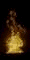FUEL TYPE
 OCTANE(C8H18)
 METHANOL(CH3OH)
 NITROMETHANE(CH3NO2)
 (LFS) m/s
 0.31
 0.43
 0.5
 DENSITY g/cc
 0.69
 0.79
 1.14
FLAME
TEMPERATURE
 1980*C
 1870*C
 2400*C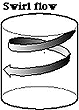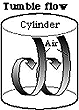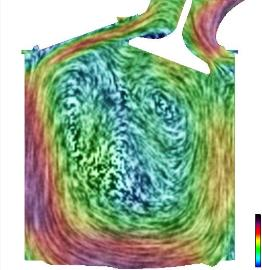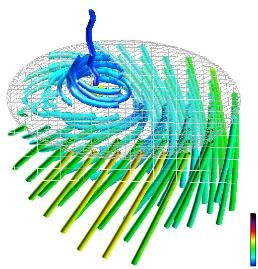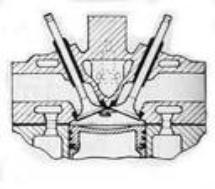FUEL TYPE
 OCTANE(C8H18)
 METHANOL(CH3OH)
 NITROMETHANE(CH3NO2)
 O2 %
 0%
 50%
 53%
 H2:CRATIO
 18%
 33%
 25%
O2:C
RATIO
 0%
 133%
 266%